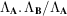International
Tables for
Crystallography
Volume B
Reciprocal space
Edited by U. Shmueli

International Tables for Crystallography (2010). Vol. B, ch. 1.3, p. 48   | 1 | 2 |

## Section 1.3.2.7.2.1. Geometric description of sublattices

G. Bricognea

aGlobal Phasing Ltd, Sheraton House, Suites 14–16, Castle Park, Cambridge CB3 0AX, England, and LURE, Bâtiment 209D, Université Paris-Sud, 91405 Orsay, France

#### 1.3.2.7.2.1. Geometric description of sublattices

| top | pdf |

Letbe a period lattice inwith matrix A, and letbe the lattice reciprocal to, with period matrix. Letbe defined similarly, and let us suppose thatis a sublattice of, i.e. thatas a set.

The relation betweenandmay be described in two different fashions: (i) multiplicatively, and (ii) additively.

 (i) We may writefor some nonsingular matrix N with integer entries. N may be viewed as the period matrix of the coarser latticewith respect to the period basis of the finer lattice. It will be more convenient to write, whereis a rational matrix (with integer determinant since det) in terms of which the two lattices are related by(ii) Call two vectors incongruent moduloif their difference lies in. Denote the set of congruence classes (or cosets') by, and the number of these classes by. The coset decomposition'representsas the disjoint union oftranslates ofis a finite lattice withelements, called the residual lattice ofmodulo. The two descriptions are connected by the relation, which follows from a volume calculation. We may also combine (i)and (ii)intowhich may be viewed as the n-dimensional equivalent of the Euclidean algorithm for integer division:is the `remainder' of the division byof a vector in, the quotient being the matrix D.